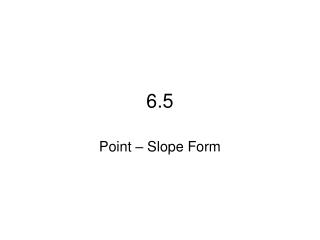DownloadDownload Presentation6.5

# 6.5

Download Presentation## 6.5

- - - - - - - - - - - - - - - - - - - - - - - - - - - E N D - - - - - - - - - - - - - - - - - - - - - - - - - - -
##### Presentation Transcript

1. 6.5 Point – Slope Form

2. Quick Review 1. What is the Slope-Intercept Form? y = mx + b 2. What is the Standard Form? Ax + By = C 3. How does an equation of a vertical line look? What is the slope? x = #, slope = undefined 4. How does an equation of a horizontal line look? What is the slope? y = #, slope = 0

3. Overview Point-Slope Form Graphing Using Point-Slope Form Writing an Equation in Point-Slope Form Writing an Equation Using a Table Forms we’ve learned so far… Slope-Intercept Form: y = mx + b Standard Form: Ax + By = C

4. Point-Slope Formy – y1 = m(x – x1) Derived (came from) the slope formula: (x1, y1) = (-2, -2) (x, y)

5. 6.5 – Point – Slope Form • Basically, when you are given a point and a slope, you can define the line by using the Point-Slope Form. y – y1 = m(x – x1) • Where m = slope and (x1, y1) is the given point.

6. Point-Slope Form 1. Write the equation in Point-Slope Form of the graph below: (x1, y1) = (-2, -2) We know the point (-2, -2), so x1 = -2 and y1 = -2 (x, y) We know the slope is 2/3 Plug it into the Point-Slope Form:

7. Graphing Using Point-Slope Form 2. Graph the equation What is the ordered pair? (x1, y1) = What is the slope? m = (2, 5) (2, 5)

8. Graphing Using Point-Slope Form 3. Graph the equation What is the ordered pair? (x1, y1) = What is the slope? m = (-2, 5)

9. Write an Equation in Point-Slope Form Given a Point and a Slope 4. (-1, -3); Slope = 4 y + 3 = 4(x + 1) 5. (-10, 0); Slope = 2 y = 2(x +10) 6. (-4, 3); Slope = -6 y – 3 = -6(x +4)

10. Write an Equation in Point-Slope Form Given Two Points Steps: • Find the slope between the two points. • Pick one point to use in order to write the equation. • Plug in the slope and coordinate from the point into the Point-Slope Form

11. 7. (-3, 6) and (1, -2) y – 6 = -2(x + 3) or y + 2 = -2(x – 1) 8. (-2, 3) and (-1, 1) y – 3 = -2(x + 2) or y – 1 = -2(x + 1) 9. (-2, -5) and (7, -6) y + 5 = - (x + 2) or y + 6 = - (x – 7) Write an Equation in Point-Slope Form Given Two Points

12. Writing an Equation from a Table What is the independent and dependent variable? x – independent; y – dependent Find the rate of change: What is the slope? 4/10 = 2/5 What is one ordered pair? (-11, -7), (-1, -3), (4, -1), (19, 5) What is a possible equation in Point-Slope Form? y + 7 = 2/5 (x + 11)

13. Wrap Up Point-Slope Form Graph Using Point-Slope form Start at the point Graph the slope Write equation in point-slope form Given a point and a slope Given two points Writing an equation from a table Find the rate of change (slope) Pick a point to plug in DLUQ: What two things are given to you in the point-slope form?